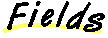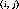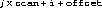`Packages  This Package  Prev  Next  Index  `

# §2.9 Class RGBImageFilter

```public  abstract  class  java.awt.image.RGBImageFilter
extends  java.awt.image.ImageFilter  (II-§2.5)
{
// Fields
protected boolean canFilterIndexColorModel;	§2.9.1
protected ColorModel newmodel;	§2.9.2
protected ColorModel origmodel;	§2.9.3

// Constructors
public RGBImageFilter();	§2.9.4

// Methods
public IndexColorModel 	§2.9.5
filterIndexColorModel(IndexColorModel  icm);
public abstract int filterRGB(int  x, int  y, int  rgb);	§2.9.6
public void filterRGBPixels(int  x, int  y, int  w,	§2.9.7
int  h, int  pixels[], int  off, int  scansize);
public void setColorModel(ColorModel  model);	§2.9.8
public void setPixels(int  x, int  y, int  w, int  h,	§2.9.9
ColorModel  model, byte  pixels[], ;int  off, int  scansize);
public void setPixels(int  x, int  y, int  w, int  h	§2.9.10
ColorModel  model, int  pixels[], int  off, int  scansize);
public void tituteColorModel(ColorModel  oldcm, 	§2.9.11
ColorModel  newcm);
}
```
This class provides an easy way to create an image filter (II-§2.5) which modifies the pixels of the original image by converting them one at a time in the default RGB color model (II-§2.1.9).

Objects of this class are meant to be used in conjunction with a filtered image source (II-§2.4) object to produce filtered versions of existing images.

This class is an abstract class. It provides the calls needed to channel all the pixel data through a single method which converts pixels, one at a time, into the default RGB color model, regardless of the color model being used by the image producer. The only method which needs to be defined to create a useable image filter is the filterRGB method.

Here is an example of a filter which swaps the red and blue components of an image:

```
class  RedBlueSwapFilter  extends  RGBImageFilter  {
public  RedBlueSwapFilter()  {
//  The  filter's  operation  does  not  depend  on  the
//  pixel's  location,  so  IndexColorModels  can  be
//  filtered  directly.
canFilterIndexColorModel  =  true;
}
public  int  filterRGB(int  x,  int  y,  int  rgb)  {
return  ((rgb  &  0xff00ff00)
|  ((rgb  &  0xff0000)  >>  16)
|  ((rgb  &  0xff)  <<  16));
}
}

```

##### canFilterIndexColorModel

```protected boolean canFilterIndexColorModel ```
Setting this value to true indicates that the the value returned by the filterRGB method (II-§2.9.6) is independent of the x and y arguments, and depends only on the rgb argument.

Subclasses of RGBImageFilter should set this field to true in their constructor if their filterRGB method does not depend on the coordinate of the pixel being filtered. Filtering the colormap entries of an indexed color map can be much faster than filtering every pixel.
The default value is false.
substituteColorModel (II-§2.9.11)
IndexColorModel (II-§2.6).

### newmodel

```protected ColorModel newmodel ```
This field is used to remember the newcm argument passed to the substituteColorModel (II-§2.9.11) method.

### origmodel

```protected ColorModel origmodel ```
This field is used to remember the oldcm argument passed to the substituteColorModel (II-§2.9.11) method.

##### RGBImageFilter

```public RGBImageFilter() ```
The default constructor.

##### filterIndexColorModel

```public IndexColorModel filterIndexColorModel(IndexColorModel icm) ```
Filters an index color model (II-§2.6) object by running each entry in its color table through the filterRGB method (II-§2.9.6). The call to filterRGB has the x and y arguments set to -1 as a flag to indicate that a color table entry is being filtered rather than an actual pixel value.

Parameters:
```icm - ```the index color model object to be filtered
Returns:
a new index color model with the filtered colors.

### filterRGB

```public abstract int filterRGB(int x, int y, int rgb) ```
Specifies a method to convert a single input pixel, whose value is specified in the default RGB color model (II-§2.1.9), to a new pixel value also in the default RGB color model. Subclasses of RGBImageFilter must provide a definition for this method.

If the value of the field canFilterIndexColorModel (II-§2.9.1) is true, then the value returned by this method must not depend on the x and y coordinates.

If the x and y arguments are both -1, this method is being called by the filterIndexColorModel method (II-§2.9.5).

Parameters:
```x - ```the x coordinate of the pixel
```y - ```the y coordinate of the pixel
```rgb - ```the value of the pixel, in the default RGB color model
Returns:
the new value of the pixel, in the default RGB color model.
filterRGBPixels (II-§2.9.7).

### filterRGBPixels

```public void filterRGBPixels(int x, int y, int w, int h, int pixels[], int off, int scansize) ```
Filters a buffer of pixels in the default RGB color model (II-§2.1.9) by passing them one by one through the filterRGB method (II-§2.9.6).

The setPixels method (II-§2.10.15) of the filter's consumer (II-§2.5.1) is then called with the resulting buffer and the color model argument set to the default RGB color model.

Only pixels that fall within the specified rectangle are modified. The value of the pixel at coordinateis stored in the pixel array at indexParameters:
```x - ```left coordinate of rectangle
```y - ```top coordinte of rectangle
```w - ```width of rectangle
```h - ```height of rectangle
```model - ```color model for bits
```pixels - ```array of bits
```off - ```offset for first element
```scansize - ```number of elements per row

### setColorModel

```public void setColorModel(ColorModel model) ```
The image producer calls the setColorModel method to specify the color model for the majority of the subsequent setPixels method calls. For more information on this method and its model argument, see §2.10.11 on page 305

The setColorModel method of RGBImageFilter determines if the color model argument is an index color model (II-§2.6) and if the canFilterIndexColorModel field (II-§2.9.1) is true.

If both conditions are true, the method creates a new color model by calling the filterIndexColorModel method (II-§2.9.5) on the model argument. The original color model and the newly created color model are then passed as arguments to the substituteColorModel (II-§2.9.11) method. In addition, the setColorModel method (II-§2.9.8) of the filter's consumer (II-§2.5.1) is called with the newly created color model.

If either condition is false, the method calls the the setColorModel method (II-§2.10.11) of its consumer (II-§2.5.1) with the default RGB color map (II-§2.1.9).

Parameters:
```model - ```a color map used in subsequent setPixel calls
Overrides:
setColorModel in class ImageFilter (II-§2.5.7).

### setPixels

```public void setPixels(int x, int y, int w, int h, ColorModel model, byte pixels[], int off, int scansize) ```
The image producer calls the setPixels method of the image consumer one or more times to deliver the pixels of the image. For more information on this method and its arguments, see §2.10.14 on page 307.

The setPixels method of RGBImageFilter looks to see if the color model is the same one that has already been converted and remembered for substitution by a previous call to the substituteColorModel (II-§2.9.11) method.

If so, it calls the setPixels method (II-§2.10.14) of its consumer (II-§2.5.1), changing the color model argument to be the alternative color model.

Otherwise, the method converts the buffer of byte pixels to the default RGB color model (II-§2.1.9) and passes the converted buffer to the filterRGBPixels (II-§2.9.7) method to be converted one by one.

Parameters:
```x - ```left coordinate of rectangle
```y - ```top coordinte of rectangle
```w - ```width of rectangle
```h - ```height of rectangle
```model - ```color model for bits
```pixels - ```array of bits
```off - ```offset for first element
```scansize - ```number of elements per row
Overrides:
setPixels in class ImageFilter (II-§2.5.10).

### setPixels

```public void setPixels(int x, int y, int w, int h, ColorModel model, int pixels[], int off, int scansize) ```
The image producer calls the setPixels method of the image consumer one or more times to deliver the pixels of the image. For more information on this method and its arguments, see §2.10.15 on page 308.

The setPixels method of RGBImageFilter looks to see if the color model is the same one that has already been converted and remembered for substitution by a previous call to the substituteColorModel (II-§2.9.11) method.

If so, it calls the setPixels method (II-§2.10.14) of the filter's consumer (II-§2.5.1), changing the color model argument to be the alternative color model.

Otherwise, the method converts the buffer of byte pixels to the default RGB color model (II-§2.1.9) and passes the converted buffer to the filterRGBPixels (II-§2.9.7) method to be converted one by one.

Parameters:
```x - ```left coordinate of rectangle
```y - ```top coordinte of rectangle
```w - ```width of rectangle
```h - ```height of rectangle
```model - ```color model for bits
```pixels - ```array of bits
```off - ```offset for first element
```scansize - ```number of elements per row
Overrides:
setPixels in class ImageFilter (II-§2.5.11).

### substituteColorModel

```public void substituteColorModel(ColorModel oldcm, ColorModel newcm) ```
Registers two color model objects for substitution: If the oldcm is the color model during any subsequent call to either of the setPixels methods (§2.9.9, §2.9.10), the newcm argument is substituted and the pixels passed through unmodifed.

Parameters:
```oldcm - ```the ColorModel object to be replaced on the fly
```newcm - ```the ColorModel object to replace oldcm on the fly

`Packages  This Package  Prev  Next  Index`
Java API Document (HTML generated by dkramer on April 22, 1996)